### Home > A2C > Chapter 10 > Lesson 10.2.6 > Problem10-150

10-150.
1. Rewrite each of the following in simplest complex or radical form. Homework Help ✎

1.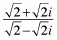2. The reciprocal of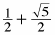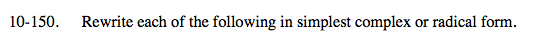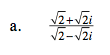$\text{Factor out the }\sqrt{2}\text{ and simplify.}$

$\frac{1+i}{1-i}$

Multiply by the conjugate of the denominator.

$\frac{(1+i)(1+i)}{(1-i)(1+i)}$

i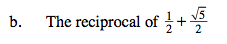See part (a).